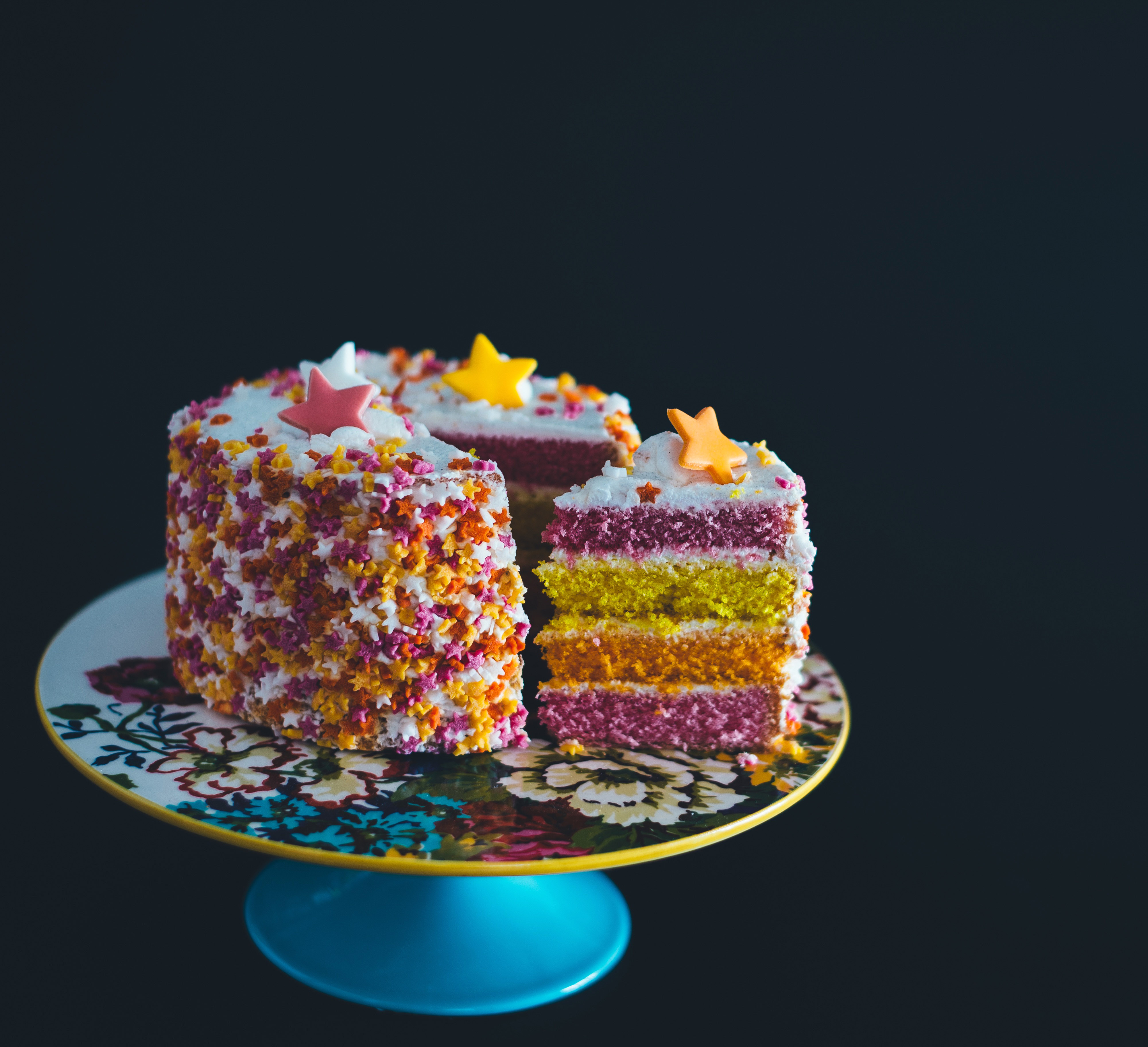## ________________

It’s Coach Newton’s birthday this week and the team celebrates by offering him some birthday math trivia and puzzles.

## ________________### Some Difference

If you add up the two-digit year that somebody was born, the number of the month of their birthday from 1 to 12, and the day of the month of their birthday, we’ll call that their “birthday sum.” For example, somebody born in 1968 on Feb. 18 would have a birthday sum of 68 + 2 + 18 = 88.

What’s the largest possible difference between birthday sums of people born on consecutive days?

One of the students jokes with the coach, “You know, you’re not really in the prime of your life.” “What do you mean?” asks Coach Newton. “Well, we noticed that the greatest number of prime ages that can occur in one round decade of your life is four, such as 2, 3, 5, and 7 in the first decade of your life, and 11, 13, 17, and 19 in your teens. Although you are near the beginning of a new decade in your life, it’s not one of those ‘prime decades’ with four prime ages.“ ”Oh, that’s OK with me,” chuckles the Coach.

What is the next prime decade after one’s teen years?

## Solutions to week 74

Frequent Figures. The problem is asking for a number which represented in one base has the form 22, 333, 4444, 55555, or so on, and in another base has a different instance of that same form. (The form “1” always represents the number one in any base, so it is not helpful.) To make the answer as small as possible, we want to use as few digits as possible. So what is 22 in base b? It represents 2×b + 2. Since b must be at least three in order to use the digit “2,” we can represent any even number greater than six as 22 in some base. So what’s the smallest even number that can be represented in another of these forms? Could it be 333 in some base? No, because if the base b is even, then 330 in base b is 3×b² + 3×b is even, so 333 is odd, being three more. And if b is odd, then 330 is 3×b² + 3×b, an odd plus an odd, so again even, and 333 is again odd. So 333 always represents an odd number in any base, and hence can’t represent the same number as 22 in some other base, since that’s always even.

So we have to try 4444. That numeral has the smallest value in base five, and it will certainly be even, so it will equal 22 in some other base. Therefore, the answer is 4444 in base five, which is 4×125 + 4×25 + 4×5 + 4 = 624.

Doubled Digit. It’s well known that any number has the same remainder when divided by nine as the sum of its digits in base ten does. For exactly the same reason, any number has the same remainder when divided by eight as the sum of its digits in base nine does. Therefore the sum of the digits of seven to the eleventh power in base nine has the same remainder when divided by eight as seven to the eleventh does. But checking the powers of seven (7, 49, 343, 2401, …) we see that their remainders when divided by eight alternate between seven and one. Therefore the remainder when seven to the eleventh power is divided by eight is seven. Thus, the sum of its digits in base nine must also have remainder seven when divided by eight. But the sum of the digits zero through eight is 36, with remainder four when divided by eight. Hence the only doubled digit that will bring the remainder to exactly seven is three.

## Recent Weeks

Links to all of the puzzles and solutions are on the Complete Varsity Math page.

Come back next week for answers and more puzzles.

[asciimathsf]